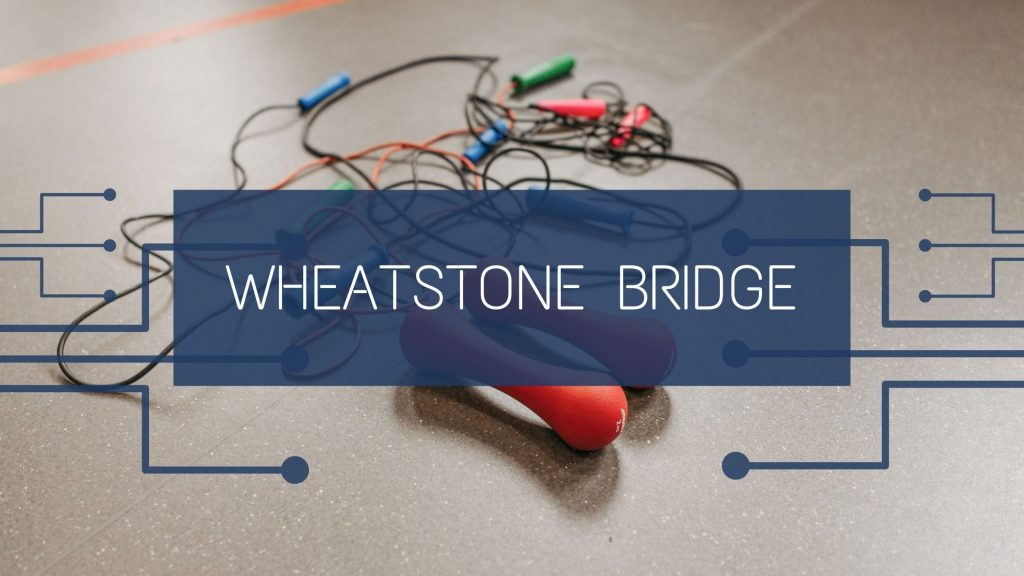Physics
Study Material

# Wheatstone Bridge- Working Principle, Formula, Diagram

Wheatstone bridge is a circuit which have two or more branches such that no current flows from resistance branch joining main branches.

3 minutes long
Posted by Mahak Jain, 29/9/2021Hesap Oluştur

Got stuck on homework? Get your step-by-step solutions from real tutors in minutes! 24/7. Unlimited.

Finding values of unknown resistances was always a problem. To overcome this problem scientists came up with the concept of Wheatstone bridge. It is primarily used to find values of unknown resistances. Along time it has found many other applications. It is another way to visualize Kirchhoff’s law.

## Principle of Wheatstone Bridge

The Wheatstone bridge works on the principle of null deflection. This means that the ratio of their resistances in one branch is equal to ratio of corresponding resistances in other branch. Thus no current flows through galvanometer. As in following diagram P/Q = R/S. We’ll derive this expression below. The bridge is in a balanced condition only and only when no current flows through the galvanometer. We can use a rheostat to achieve this condition.

## Wheatstone Bridge Formula

Formula of wheatstone bridge simply is the ratio of resistances. Thus, from the above figure,

P/Q = R/S

Or, P/R = Q/S

## Wheatstone Bridge Derivation

As an application of Kirchhoff’s rules consider the circuit shown in figure, which is the Wheatstone bridge. The bridge has four resistors R1, R2, R3 and R4. Across one pair of diagonally opposite points (A and C in the figure) a source is connect. This (i.e., AC) is the battery arm. Between the other two vertices, B and D, a galvanometer G (which is a device to detect currents) is connected. This line, shown as BD in the figure, is called the galvanometer arm. For simplicity, we assume that the cell has no internal resistance.

In general there will be currents flowing across all the resistors as well as a current Ig through G. Of special interest, is the case of a balanced bridge where the resistors are such that Ig = 0. We can easily get the balance condition, such that there is no current through G. In this case, the Kirchhoff’s junction rule applied to junctions D and B (see the figure) immediately gives us the relations I1 = I3 and I2 = I4. Next, we apply Kirchhoff’s loop rule to closed loops ADBA and CBDC. The first loop gives

R2/R1 = R3/R4

This last equation relating the four resistors is the balance condition for the galvanometer to give zero or null deflection. The Wheatstone bridge and its balance condition provide a practical method for determination of an unknown resistance. Let us suppose we have an unknown resistance, which we insert in the fourth arm; R4 is thus not known. Keeping known resistances R1 and R2 in the first and second arm of the bridge, we go on varying R3 till the galvanometer shows a null deflection.